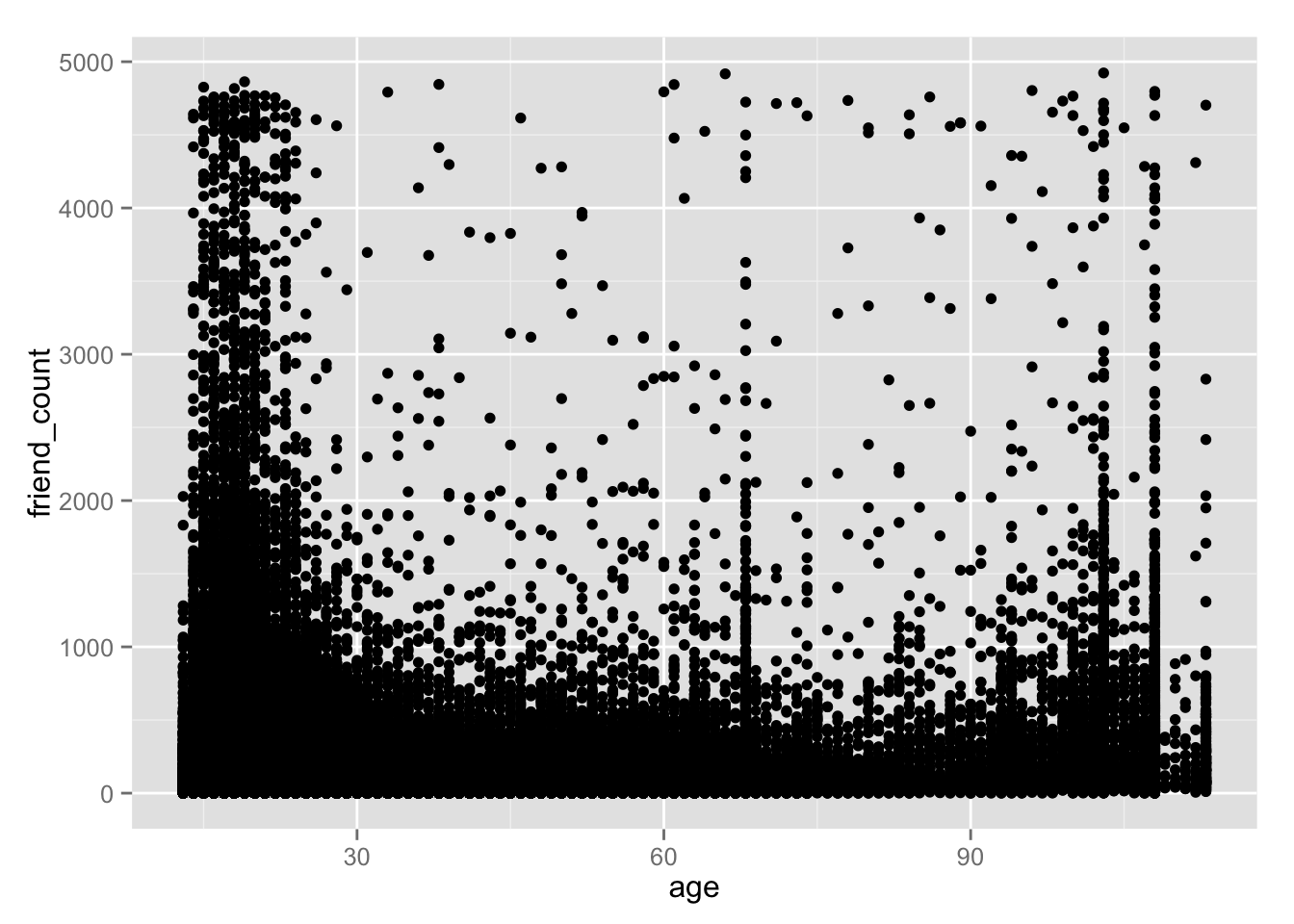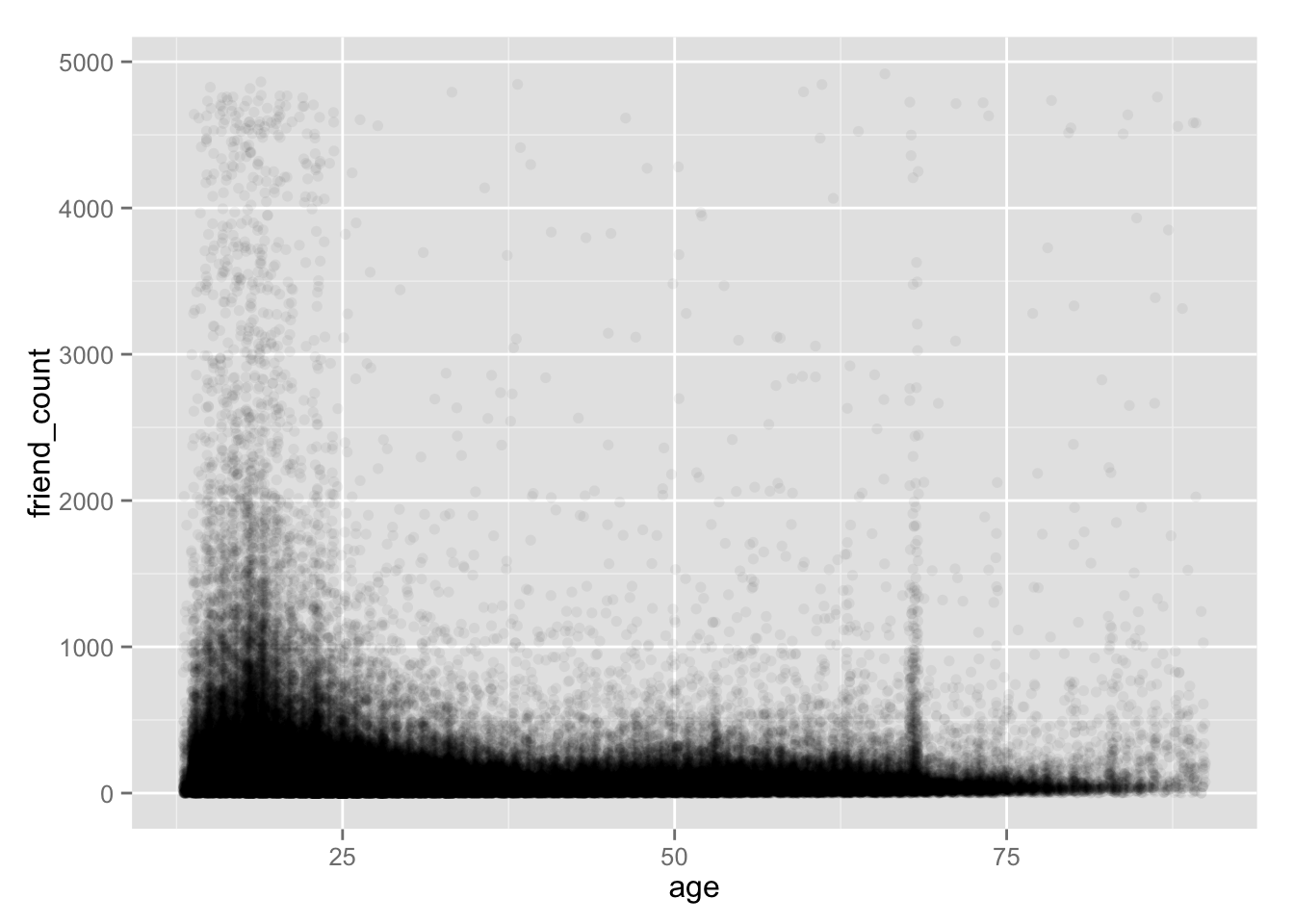# exploring-two-variables

|   Source

Exploring two variables in R with scatterplot, jitter and smoothing to handle overplotting

In this lesson we will learn how toInvestigate two variable make a Scatter Plot and hear moira’s study in EDA perceive audience size ### Scatterplots and Perceived Audience Size Notes: x->actual vs y->perceive. We can see that people choose round up number(50,100,200,etc) when they perceived audience size In reality, people saw our post saw 100/200 ***

### Scatterplots

Notes:

``````library(ggplot2)
ggplot(aes(x = age, y=friend_count), data = pf) + geom_point()``````#### What are some things that you notice right away?

Response: People below thirty would have more friends.there’s some extreme where ages>90 (some maybe lying). But that also can infer people who fake beyond age 90 have sense of humor hence more friends. It’s also important to notice the outliers of our data, and make actions how to audit the data. ***

### ggplot Syntax

Notes: Need to say aes wrapper in x and y have to say what type of geom

``summary(pf\$age)``
``````##    Min. 1st Qu.  Median    Mean 3rd Qu.    Max.
##   13.00   20.00   28.00   37.28   50.00  113.00``````
``ggplot(aes(x = age, y=friend_count), data = pf) + geom_point() ``### Overplotting

Notes: Overplotting means we can’t exactly see what are the real plotting. In this case we want to have a plot represent on 20 plot As X(age) is discrete, only attribute point doesn’t really describe age. So instead we using jitter The warning of ommited missing values because we limit to only age 13-90

``ggplot(aes(x = age, y=friend_count), data = pf) + geom_jitter(alpha=1/20) + xlim(13,90)``
``## Warning: Removed 5176 rows containing missing values (geom_point).``#### What do you notice in the plot?

Response: We can see more distributed in the plot. Also keep in mind alpha=1/20 in geom means it will take 20 points in that coordinat to make it completely black. By doing this we know that most of users(in block of black) seen as age over 30 has below 1000 average friends. ***

### Coord_trans()

Notes:

``````ggplot(aes(x = age, y=friend_count), data = pf) +
geom_point(alpha=1/20,position= position_jitter(h = 0)) +
xlim(13,90)``````
``## Warning: Removed 5177 rows containing missing values (geom_point).``